NEET  >  Test: Common Forces in Mechanics (NCERT)

# Test: Common Forces in Mechanics (NCERT)

Test Description

## 15 Questions MCQ Test Physics Class 11 | Test: Common Forces in Mechanics (NCERT)

Test: Common Forces in Mechanics (NCERT) for NEET 2023 is part of Physics Class 11 preparation. The Test: Common Forces in Mechanics (NCERT) questions and answers have been prepared according to the NEET exam syllabus.The Test: Common Forces in Mechanics (NCERT) MCQs are made for NEET 2023 Exam. Find important definitions, questions, notes, meanings, examples, exercises, MCQs and online tests for Test: Common Forces in Mechanics (NCERT) below.
Solutions of Test: Common Forces in Mechanics (NCERT) questions in English are available as part of our Physics Class 11 for NEET & Test: Common Forces in Mechanics (NCERT) solutions in Hindi for Physics Class 11 course. Download more important topics, notes, lectures and mock test series for NEET Exam by signing up for free. Attempt Test: Common Forces in Mechanics (NCERT) | 15 questions in 15 minutes | Mock test for NEET preparation | Free important questions MCQ to study Physics Class 11 for NEET Exam | Download free PDF with solutions
 1 Crore+ students have signed up on EduRev. Have you?
Test: Common Forces in Mechanics (NCERT) - Question 1

### Which of the following statements is correct about friction?

Detailed Solution for Test: Common Forces in Mechanics (NCERT) - Question 1
• The coefficient of friction between a given pair of substances is independent of the area of contact between them.
• μ > 1 for copper on cast iron.
• Rolling friction is much smaller than sliding friction. Irregularity of the surfaces in contact is not a main contributor of friction
Test: Common Forces in Mechanics (NCERT) - Question 2

### Identify the correct statement.

Detailed Solution for Test: Common Forces in Mechanics (NCERT) - Question 2
• Both static and kinetic friction are independent on the area of contact.
• Coefficient of static friction depends on the surfaces in contact. μk < μs
Test: Common Forces in Mechanics (NCERT) - Question 3

### Which of the following is a self adjusting force?

Detailed Solution for Test: Common Forces in Mechanics (NCERT) - Question 3

Static friction is a self adjusting force.

Test: Common Forces in Mechanics (NCERT) - Question 4

Which one of the following can also act as a lubricant in machines?

Detailed Solution for Test: Common Forces in Mechanics (NCERT) - Question 4

In the machines, the flow of compressed and purified air lowers the friction.
Hence it acts as a lubrican

Test: Common Forces in Mechanics (NCERT) - Question 5

A girl presses her physics textbook against a rough vertical wall with her hand. The direction of the frictional force on the book exerted by the wall is:

Detailed Solution for Test: Common Forces in Mechanics (NCERT) - Question 5

Friction forces are always parallel to the surfaces in contact, which in this case, are the wall and the cover of the book. This tells us that the friction force is either upwards or downwards. Because the tendency of the book is to fall due to gravity, the friction force must be in the upwards direction.

Test: Common Forces in Mechanics (NCERT) - Question 6

A car accelerates on a horizontal road due to the force exerted by

Detailed Solution for Test: Common Forces in Mechanics (NCERT) - Question 6

A car accelerates on a horizontal road due to the force exerted by the road on the car.

Test: Common Forces in Mechanics (NCERT) - Question 7

A block of mass m rests on a rough inclined plane. The coefficient of friction between the surface and the block is µ. At what angle of inclination theta of the plane to the horizontal will the block just start to slide down the plane?

Detailed Solution for Test: Common Forces in Mechanics (NCERT) - Question 7

The various forces acting on the block are as shown in the figure. From figure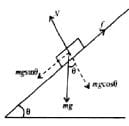mgsinθ = f     ....(i)
​mgcosθ = N     ....(i)
Divide (i) by (ii), we get
tanθ =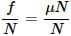or
θ = tan−1(μ)

Test: Common Forces in Mechanics (NCERT) - Question 8

When a body slides down from rest along a smooth inclined plane making an angle of 30 with the horizontal, it takes time 20s. When the same body slides down from rest along a rough inclined plane making the same angle and through the same distance, it takes time 20p s, where p is some number greater than 1. The coefficient of friction between the body and the rough plane is

Detailed Solution for Test: Common Forces in Mechanics (NCERT) - Question 8

The acceleration of the body sliding down smooth inclined plane is
a = gsinθ …(i)
The acceleration of the same body sliding down a rough inclined plane is
a′ = gsinθ − μgcosθ
= g (sinθ−μcosθ) ...(ii)
As the body slides a distance d in each case,
∴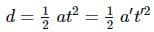(∵ u = 0)
∴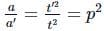(∵ t′ = 20ps,t=20s)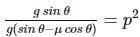(Using (i) and (ii))

sinθ = p2sinθ − μp2 cosθ; μp2 cosθ
= p2sinθ − sinθ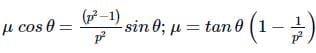Here, θ=30∘ ∴ μ =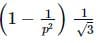Test: Common Forces in Mechanics (NCERT) - Question 9

The minimum force required to start pushing a body up a rough (frictional coefficient μ) inclined plane is F1 while the minimum force needed to prevent it from sliding down is F2. If the inclined plane makes an angle θ from the horizontal such that tan θ = 2μ then the ratio F1/F2 is :

Detailed Solution for Test: Common Forces in Mechanics (NCERT) - Question 9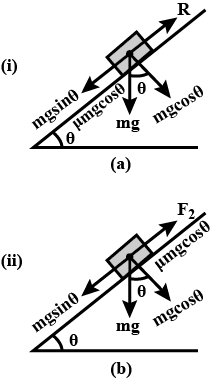The minimum force required to start pushing a body up a rough inclined plane is F= mgsinθ + μgcosθ  ......(i)
Minimum force needed to prevent the body from sliding down the inclined plane is F2 = mgsinθ − μgcosθ     .....(ii)
Divide (i) by (ii), we get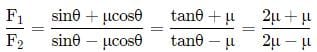= 3 (tanθ=2μ (given))

Test: Common Forces in Mechanics (NCERT) - Question 10

A block of mass 10kg is placed on rough horizontal surface whose coefficient of friction is 0.5. If a horizontal force of 100N is applied on it, then acceleration of the block will be(Take g = 10 m s-2)

Detailed Solution for Test: Common Forces in Mechanics (NCERT) - Question 10

Here, m = 10 kg, g= 10 m s-2, μ = 0.5, F = 100N
Force of friction
f = μN = μMg = (0.5)(10)(10) = 50N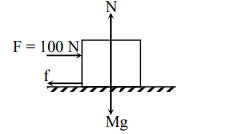Force  that produce acceleration
F' = F - f = 100 N - 50 N = 50 N
a = F'/ m = 50 N / 10 kg = 5 m s-2

Test: Common Forces in Mechanics (NCERT) - Question 11

The coefficient of static friction between the box and the train's floor is 0.2. The maximum acceleration of the train in which a box lying on its floor will remain stationary is (Take g = 10 ms−2)

Detailed Solution for Test: Common Forces in Mechanics (NCERT) - Question 11

As acceleration of the box is due to static friction,
∴ ma = fs ≤ μs N = μs mg
a ≤ μsg
∴ amax  = μg = 0.2 × 10ms−2
= 2ms−2

Test: Common Forces in Mechanics (NCERT) - Question 12

A block of mass 1 kg lies on a horizontal surface in a truck. The coefficient of static friction between the block and the surface is 0.6. If the acceleration of the truck is 5ms−2. The frictional force acting on the block is then

Detailed Solution for Test: Common Forces in Mechanics (NCERT) - Question 12

Limiting friction, f = μmg = 0.6 × 1 × 9.8
= 5.88 N
Applied force, F = ma = 1 × 5 = 5N
As F < f, so force of friction = 5 N

Test: Common Forces in Mechanics (NCERT) - Question 13

A block of mass 2kg rest on a plane inclined at an angle of 30 with the horizontal. The coefficient of friction between the block and the surface is 0.7. What will be the frictional force acting on the block?

Detailed Solution for Test: Common Forces in Mechanics (NCERT) - Question 13

Here, frictional force, f = μR
= μmgcosθ = 0.7 × 2 × 9.8cos30
= 0.7 × 2 × 9.8 × 0.866 = 11.9 N

Test: Common Forces in Mechanics (NCERT) - Question 14

A block of mass M is held against a rough vertical wall by pressing it with a finger. If the coefficient of friction between the block and the wall is μ and the acceleration due to gravity is g, what is the minimum force required to be applied by the finger to hold the block against the wall?

Detailed Solution for Test: Common Forces in Mechanics (NCERT) - Question 14

Let F be the force applied by the finger on the block. N is normal reaction of the wall on the block. Mg is weight of block acting vertically downwards. f is the force of friction between the wall and the block which acts upwards as shown in the figure.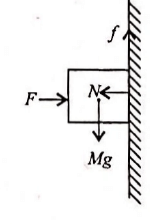The block will not fall, if f = Mg
μN = Mg  (∵ f = μN)
μF = Mg (∵ N = F)  = F = Mg/μ

Test: Common Forces in Mechanics (NCERT) - Question 15

A trolley of mass 20kg is attached to a block of mass 4kg by a massless string passing over a frictionless pulley as shown in the figure. If the coefficient of kinetic friction between trolley and the surface is 0.02, then the acceleration of the trolley and block system is(Take g = 10ms−2)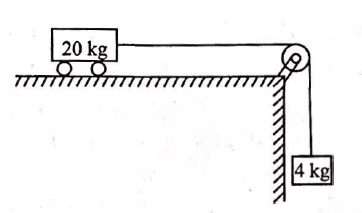Detailed Solution for Test: Common Forces in Mechanics (NCERT) - Question 15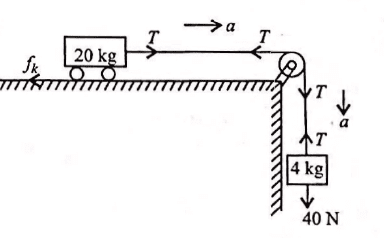As the string is inextensible, and the pulley is smooth, therefore both the 4kg block and the 20kg trolley have the same acceleration a.
Let T be tension in the string.
The equation of motion of the block is
40 − T = 4a  …(i)
The equation of motion of the trolley is
T − fk  = 20a
fk = μk N

Here, μk =0.02, N = 20 × 10 = 200N

∴ T − 0.02 × 200 = 20a or
T − 4 = 20a …(ii)
Adding (i) and (ii), we get,
a = 36 / 24 m s−2  = 1.5 m s−2

## Physics Class 11

127 videos|464 docs|210 tests
 Use Code STAYHOME200 and get INR 200 additional OFF Use Coupon Code
Information about Test: Common Forces in Mechanics (NCERT) Page
In this test you can find the Exam questions for Test: Common Forces in Mechanics (NCERT) solved & explained in the simplest way possible. Besides giving Questions and answers for Test: Common Forces in Mechanics (NCERT), EduRev gives you an ample number of Online tests for practice

## Physics Class 11

127 videos|464 docs|210 tests

### How to Prepare for NEET

Read our guide to prepare for NEET which is created by Toppers & the best Teachers